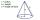Decide

The rectangle is divided into seven fields. On each box is to write just one of the numbers 1, 2 and 3.

Mirek argue that it can be done so that the sum of the two numbers written next to each other was always different. Zuzana (Susan) instead argue that it is not possible. Decide which of them is right.

Result

Leave us a comment of this math problem and its solution (i.e. if it is still somewhat unclear...):Be the first to comment!Next similar math problems:

1. Street numbersLada came to aunt. On the way he noticed that the houses on the left side of the street have odd numbers on the right side and even numbers. The street where he lives aunt, there are 5 houses with an even number, which contains at least one digit number 6.
2. Write 2Write 791 thousandths as fraction in expanded form.
3. Unknown number 11That number increased by three equals three times itself?
4. Round it0.728 round to units, tenths, hundredths.
5. The diagramThe diagram is a cone of radius 8cm and height 10cm. The diameter of the base is. ..
6. Cupcakes 2Susi has 25 cupcakes. She gives 4/5. How much does she have left?
7. Pizza 4Marcus ate half pizza on monday night. He than ate one third of the remaining pizza on Tuesday. Which of the following expressions show how much pizza marcus ate in total?
8. Simple equation 9Solve the following equation: -8y+5=-9y+9
9. Simple equationSolve the following simple equation: 2. (4x + 3) = 2-5. (1-x)
10. Negative in equation2x + 3 + 7x = – 24, what is the value of x?
11. Dropped sheetsThree consecutive sheets dropped from the book. The sum of the numbers on the pages of the dropped sheets is 273. What number has the last page of the dropped sheets?
12. MO 2016 Numerical axisCat's school use a special numerical axis. The distance between the numbers 1 and 2 is 1 cm, the distance between the numbers 2 and 3 is 3 cm, between the numbers 3 and 4 is 5 cm and so on, the distance between the next pair of natural numbers is always in
13. DisjointHow many elements have union and intersection of two disjoint sets when the first have 1 and secodn 8 elements.
14. WithdrawalIf I withdrew 2/5 of my total savings and spent 7/10 of that amount. What fraction do I have in left in my savings?
15. Passenger boatTwo-fifths of the passengers in the passenger boat were boys. 1/3 of them were girls and the rest were adult. If there were 60 passengers in the boat, how many more boys than adult were there?
16. AlleyAlley measured a meters. At the beginning and end are planted poplar. How many we must plant poplars to get the distance between the poplars 15 meters?
17. RoundingThe following numbers round to the thousandth: